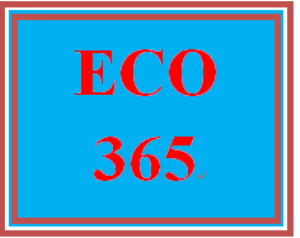# ECO 365T Wk 5 - Practice Knowledge Check (2021 New)

PLDZ-16135 Free In Stock
\$ 0.00 USD
Description

http://www.onlinehelp123.com/ECO-365

~~~~~~~~~~~~~~~~~~~~~~~~~~~~~~

http://www.onlinehelp123.com/ECO-365

ECO 365T Wk 5 - Practice Knowledge Check (2021 New)

Refer to the provided graph showing the marginal product (MPL) and the average product of labor (APL). At which quantity of labor employed is total product maximized?

Multiple Choice

•      A
•      B
•      C
•      D

(Consider This) Which of the following is an example of a sunk cost, as it relates to a firm?

Multiple Choice

•      an expenditure on raw materials used in the production process
•      an expenditure on a nonrefundable, nontransferable airline ticket
•      an expenditure to buy a delivery van
•      an expenditure for a new factory

If the total cost of 20 units of a product is \$20, and the total cost of 21 units is \$21, then from 20 to 21 units of product the

Multiple Choice

•      marginal cost is decreasing.
•      marginal cost equals average total cost.
•      marginal cost equals average variable cost.
•      average total cost equals average variable cost.

Refer to the graph. Which one of the following would cause a move from point d to point e along short-run average total cost curve ATC2?

Multiple Choice

•      diminishing marginal returns
•      an increase in the wage rate
•      a decrease in the wage rate
•      increasing marginal returns

Answer the question on the basis of the following output data for a firm. Assume that the amounts of all nonlabor resources are fixed.

Number of Workers       Units of Output

0     0

1     40

2     90

3     126

4     150

5     165

6     180

What is the firm’s average product when three workers are hired?

Multiple Choice

•      18 units of output.
•      36 units of output.
•      42 units of output.
•      21 units of output.

In the short run, total output in an industry

Multiple Choice

•      is fixed at a specific level.
•      can vary as the result of using a fixed amount of plant and equipment more or less intensively.
•      may be altered by varying the size of plant and equipment which now exist in the industry.
•      can vary as the result of new firms entering or leaving the industry.

Tags
Recent Reviews Write a Review
0 0 0 0 reviews